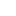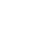# Determination of conductance of a strong acid (HCl)

Determination of conductance of a strong acid And Weak acid (HCl)

#### Chemicals Required:

The chemicals required are:

• Acetic Acid
• Hydro Chloric Acid
• Distilled water

#### Glassware:

Required Glasswares:

• 2 Beakers(100&250 ml)
• A Pipette (10ml)
• A Measuring cylinder (50 ml)

#### Apparatus:

Required apparatus:

• A Conductivity meter
• An Electrical Balance
• Iron Stand
• Clamp

#### Theory:

Conductance is an expression of these ions due to which current flows through a substance. Conductance is shown by an upper case letter “G”.
The reciprocal of resistance is called conductance.
Conductance= 1/Resistance
The units used for conductance are ohm-1 or mho. Its unit is also known as Siemens.

#### Siemens:

It can be defined as:
If a current of one ampere passes through a conductor/ mixture across which a voltage of one volt exists then conductance is known as one Siemens.

#### Factors of Conductance:

The conductance of solution is due to:

• Number of ions
• Movements of ions

In general, when the applied voltage is held constant, the current In a DC is directly proportional to the conductance. When the numbers of ions increase the conductance due to ions also increases.

#### Conductivity meter:

An electrical conductivity meter measures the electrical conductivity in a solution in ionic forms. Basically, movements of ions produce the conduction. The conductivity meter has two modes.

• Conductance mode
• Resistance mode

Conductometry is often applied to determine the total conductance of a solution or to analyze the endpoint of titration that includes ions. A conductivity meter or Conductometry has a sensor in the electrodes that measure the conductance.

Determination of conductance of a strong acid And Weak acid (HCl)

#### Principle:

Conductivity meter which measures the conductivity, two plates are placed in the sample solution and a potential is applied across the plates i.e. normally a sine wave voltage, and the current that passes through the solution is measured.

#### Calibrations of conductivity meter:

There are some manufacturing faults in equipment which lead to wrong readings and further calculations.
So calibration is required for correct and accurate readings. Following steps are necessary to calibrate a conductivity meter, which is easy and standardized:

• The conductivity meter usually contains a menu item that allows to enter the calibration mode
• Change the setting on the side that can be adjusted with a small screwdriver or tool
• Now place the probe in a solution of known conductivity value and temperature and set the meter to that conductivity

#### Procedure:

• Glassware which was used in this experiment was washed with detergents to remove the impurities and rinsed first with tap water then with distilled water and dried properly
• Solutions of different concentrations of HCl and CH3COOH were prepared
• Conductometer was calibrated by dipping its electrode in distilled water. It was managed at conductance mode
• First of all the conductance of different concentrations of weak electrolyte i.e. CH3COOH was measured
• For this purpose 50ml of CH3COOH solution of 0.1M was taken in a beaker and an electrode of Conductometer was dipped in it and noticed the readings
• The same procedure was repeated for all concentrations of CH3COOH required for the experiment
• Now the conductance of HCl solutions of all concentrations was found one by one
• All readings were taken separately

Determination of conductance of a strong acid And Weak acid (HCl)

#### Observations and calculations:

Solution preparation:
Required concentrations of weak electrolyte
(CH3COOH)
Required concentrations of CH3COOH

• C1=0.1M
• C2=0.2M
• C3=0.3M
• C4=0.4M
• C5=0.5M

Required Weight =molarity× molecular weight×
Amount of compound = V/1000
Amount of compound = 0.1 × 60 × 100 /1000
Amount of compound = 0.6 g
Basically, acetic acid (CH3COOH) is available in solution form or liquid form. So we need to find out the volume.
Density of acetic acid = 1.05 g cm-3
Purity of acetic acid = 100%
Required Volume = Mass / Density
Required Volume = 60 / 1.05
Required Volume = 57.14 ml
So we need the volume for 0.6 g
As we know
60 g of acetic acid contains by volume = 57.14 ml
1 g of acetic acid contains by volume = 57.14 ml / 60
0.6g of acetic acid contains by volume =57.14 ml/60 × 0.6
= 0.6 ml
Because acetic acid is 100% pure so 0.6 ml of acetic acid was taken from the reagent bottle with the help of a measuring cylinder and poured into a 100 ml measuring flask and diluted with distilled water up to the mark.
Similarly, all required concentrations were prepared with the same procedure.
Required concentrations of strong electrolyte
(HCl)
C1=0.1M
C2=0.2M
C3=0.3M
C4=0.4M
C5=0.5M
Concentrations of (HCl)
C1

Percentage purity of HCl=31.5%
Density of HCl=1.16g/cm3

Amount of HCl in 1M solution in 1000ml=36.5g
Amount of HCl in 1M solution in 1ml=36.5/1000 g
Amount of HCl in 1M solution in 100ml=36.5×100/1000g
Amount of HCl in 0.1M solution in 100ml=3.65×0.1g
=0.365g
HCl is always available in solution form, so we have to find the volume of HCl.

Volume= Mass/Density
= 0.365/1.16
= 0.314ml

As solution of HCl is not 100% so further calculations must be carried out
Purity of HCl solution= 31.5%
So
31.5ml of HCl are present in = 100l
1ml of HCl is present in = 100/31.5ml
0.314ml of HCl is present in = 100×0.314/51.5ml
= 0.99ml = 1ml
So 1ml of HCl was taken with the help of a measuring cylinder and poured into a 100ml measuring flask and then filled the flask with distilled water up to the mark.
Similarly, all required concentrations were formed in a similar way.

#### Conductance of CH3COOH

 Sr.no Concentration Conductance (Siemens) 1 O.1 1.5×104 2 O.2 1.6×104 3 0.3 1.7×104 4 0.4 1.9×104 5 0.5 2.1×104

#### Conductance of HCl

 Sr.no Concentration (M) Conductance (Siemens) 1 O.1 2.1×104 2 O.2 2.3×104 3 O.3 2.5×104 4 O.4 2.8×104 5 O.5 3.1×104

#### Result:

The conductance of a strong electrolyte is greater than the conductance of a weak electrolyte with a respective concentration.
The conductance increases with an increase in the molarity of an electrolyte.

#### Precautions:

• Make solutions carefully because both acids can burn the skin if spit
• Do not use tap water while making solutions of different concentration because tap water contains different ions which affects the concentrations, always use distilled water
• Try to use a little number of chemicals and perform the experiment at lower concentrations
• Avoid eating and drinking activities during lab performance
• Gloves and glasses are necessary during lab experiments
• Avoid horseplay during a lab experiment

#### See All Practical###### Don’t miss these Chemistry topics!
Sharing Is Caring:

### 7 thoughts on “Determination of conductance of a strong acid (HCl)”

1.Nice work. It’s really very helpful for me to perform and write practical easily. I don’t need to stray at different websites for even a single reliable definition… But here are all of these.
I liked your work and appreciate you

•thanks

•My pleasure

2.AMAZING

•thanks

3.Comprehensive description of the subject matter
Fine effort
Keep it up

•